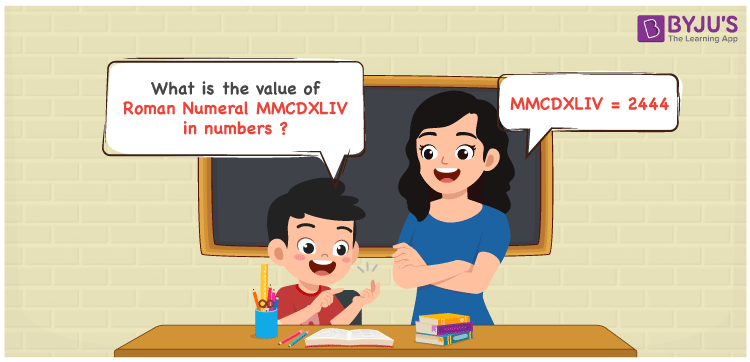Checkout JEE MAINS 2022 Question Paper Analysis : Checkout JEE MAINS 2022 Question Paper Analysis :

# MMCDXLIV Roman Numerals

MMCDXLIV Roman Numerals is 2444. In this article, MMCDXLVI is expanded according to the place value and then is converted to numeric form. Students should pay more attention to these concepts to score good marks. Roman numerals articles give a brief explanation of the minute concepts which have to be understood well during the conversion. Therefore, MMCDXLIV can be indicated as 2444 in numbers.

 Number Roman Numeral 2444 MMCDXLIV## How to Write MMCDXLIV Roman Numerals in Numbers?

Learn how MMCDXLIV is indicated in numeric form by going through the steps below.

MMCDXLIV = M + M + (D – C) + (L – X) + (V – I)

MMCDXLIV = 1000 + 1000 + (500 – 100) + (50 – 10) + (5 – 1)

MMCDXLIV = 2444

## Frequently Asked Questions on MMCDXLIV Roman Numerals

### Write the roman numerals of MMCDXLIV.

We know that

MMCDXLIV = M + M + (D – C) + (L – X) + (V – I)

MMCDXLIV = 1000 + 1000 + (500 – 100) + (50 – 10) + (5 – 1)

MMCDXLIV = 2444

### What is the value of 2500 – 56?

We know that

2500 + 56 = 2444

Hence, the value of 2500 + 56 is 2444 which is written as MMCDXLIV.

### What is the remainder we obtain when MMCDXLIV is divided by XVI?

We know that

MMCDXLIV = 2444

XVI = 16

So the remainder we obtain when MMCDXLIV is divided by XVI is XII.